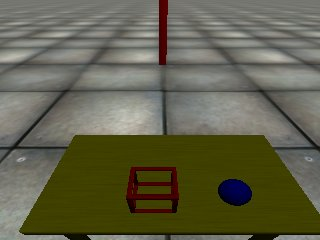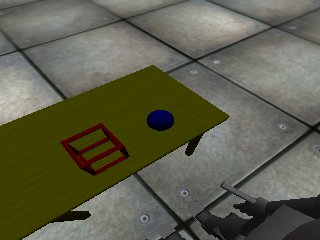iCub-main
Basic Image Processing

# Goal

To choose a visual target and fixate it. For simplicity, the target shall be "blueish stuff", and we'll work on the simulator.

So if the simulated robot sees something like this:# Programs to write

Let's make two programs:

• find_location.cpp, this will look at the input from a camera and decide what location within it looks interesting
• look_at_location.cpp, this will take an image location and try to move the camera to look towards it

For the first program, find_location, we can use any camera. For the second, we need to use the camera on the robot or the robot simulator. With the simulator, use the "world on" flag in the activation configuration file (conf/iCub_parts_activation.ini) so that the robot has a blue ball on a table in front of it. At the time of writing, you can move the robot's eyes down to see the ball by running:

and typing:

set pos 0 -60

(this should turn the eyes 60 degrees downward).

# Find Location

In YARP, camera images are sent from one program to another using ports. In C++, we can create a port for reading or writing images like this:

BufferedPort<ImageOf<PixelRgb> > port;

This means "make a port with buffering for sending/receiving images in RGB format".

Here's a basic program to get images repeatedly:

#include <stdio.h>
/* Get all OS and signal processing YARP classes */
#include <yarp/os/all.h>
#include <yarp/sig/all.h>
using namespace yarp::os;
using namespace yarp::sig;
int main() {
Network yarp; // set up yarp
BufferedPort<ImageOf<PixelRgb> > imagePort; // make a port for reading images
imagePort.open("/tutorial/image/in"); // give the port a name
while (1) {
if (image!=NULL) { // check we actually got something
printf("We got an image of size %dx%d\n", image->width(), image->height());
}
}
return 0;
}

You can compile this any way you like. One quick way to do it is to go into the directory where you saved this program (in a file called for example "find_location.cpp") and type:

yarp cmake

This creates a basic CMakeLists.txt project file that is good enough to compile most simple programs. Run cmake in this directory, and compile. You will get a program called "yarpy" (you can and should change this name by editing the CMakeLists.txt file). If you run it, you should see a port called "/tutorial/image/in" being created.

You can then connect an image source to that port using:

yarp connect <name of image port> /tutorial/image/in

for example, for the simulator, it would be something like:

yarp connect /icubSim/cam/left /tutorial/image/in

you should now see messages like this:

```  We got an image of size 320x240
We got an image of size 320x240
We got an image of size 320x240
...
```

Now let's extend our program a bit, to pick up blueish objects (for example):

#include <stdio.h>
// Get all OS and signal processing YARP classes
#include <yarp/os/all.h>
#include <yarp/sig/all.h>
using namespace yarp::os;
using namespace yarp::sig;
int main() {
Network yarp; // set up yarp
BufferedPort<ImageOf<PixelRgb> > imagePort; // make a port for reading images
imagePort.open("/tutorial/image/in"); // give the port a name
while (1) { // repeat forever
if (image!=NULL) { // check we actually got something
printf("We got an image of size %dx%d\n", image->width(), image->height());
double xMean = 0;
double yMean = 0;
int ct = 0;
for (int x=0; x<image->width(); x++) {
for (int y=0; y<image->height(); y++) {
PixelRgb& pixel = image->pixel(x,y);
// very simple test for blueishness
// make sure blue level exceeds red and green by a factor of 2
if (pixel.b>pixel.r*1.2+10 && pixel.b>pixel.g*1.2+10) {
// there's a blueish pixel at (x,y)!
// let's find the average location of these pixels
xMean += x;
yMean += y;
ct++;
}
}
}
if (ct>0) {
xMean /= ct;
yMean /= ct;
}
if (ct>(image->width()/20)*(image->height()/20)) {
printf("Best guess at blue target: %g %g\n", xMean, yMean);
}
}
}
return 0;
}

Now that we have a target, let's output it. We can output it as a YARP Vector for example, using a port like this:

BufferedPort<Vector> targetPort;

We add some lines at the beginning of the program:

Network yarp;
BufferedPort<ImageOf<PixelRgb> > imagePort;
BufferedPort<Vector> targetPort; // ADD THIS LINE

and then when we know what our target is, we send it:

printf("Best guess at blue target: %g %g\n", xMean, yMean);
Vector& v = targetPort.prepare();
v.resize(3);
v = xMean;
v = yMean;
v = 1; // a confidence value, always good practice to add. In this case, we pretend to be very confident
targetPort.write(); // send our data

When no target is selected, it is still good to send output, to say explicitly that there is no output rather than just remaining silent (which is hard to distinguish from the program just stopping or not running).

/* dd this for the condition where no target is picked */
Vector& v = targetPort.prepare();
v.resize(3);
v = 0;
v = 0;
v = 0;
targetPort.write(); // send our data

Now we can read from this port (just to test) with yarp read:

# Look at Location

Now let's write a separate program that takes the target location computed in find_location.cpp and drives the real of simulated camera to look towards that location. First, we need to read our vector:

#include <cstdio>
#include <vector>
#include <yarp/os/all.h>
#include <yarp/sig/all.h>
using namespace std;
using namespace yarp::os;
using namespace yarp::sig;
int main() {
Network yarp; // set up yarp
BufferedPort<Vector> targetPort;
targetPort.open("/tutorial/target/in");
while (1) { // repeat forever
if (target!=NULL) { // check we actually got something
printf("We got a vector of size %d\n", target->size());
}
}
return 0;
}

Compile using the same method as before. If we run the two programs, connect an image source to the first one, and do:

yarp connect /tutorial/target/out /tutorial/target/in

We should see messages like this:

```  We got a vector of size 3
We got a vector of size 3
...
```

Let's change our message from:

printf("We got a vector of size %d\n", target->size());

to:

printf("We got a vector containing");
for (int i=0; i<target->size(); i++) {
printf(" %g", (*target)[i]);
}
printf("\n");

We should see messages like this:

```  We got a vector containing 234.459 191.892 1
We got a vector containing 234.459 191.892 1
We got a vector containing 234.459 191.892 1
...
```

or

```  We got a vector containing 0 0 0
We got a vector containing 0 0 0
We got a vector containing 0 0 0
```

if no target is visible.

Now we need to drive the motors (see Getting accustomed with motor interfaces). For this, we need the YARP device classes, so we add another header file at the start of our program:

#include <yarp/dev/all.h>
using namespace yarp::dev;

We connect to the simulator head:

Property options;
options.put("device", "remote_controlboard");
options.put("local", "/tutorial/motor/client");
return 1;
}
IControlMode *mod;
IPositionControl *pos;
IVelocityControl *vel;
IEncoders *enc;
if (mode==NULL || pos==NULL || vel==NULL || enc==NULL) {
printf("Cannot get interface to robot head\n");
return 1;
}
int jnts = 0;
pos->getAxes(&jnts);
Vector setpoints;
setpoints.resize(jnts);
// enable velocity control mode
vector<int> modes(jnts,VOCAB_CM_VELOCITY);
mod->setControlModes(modes.data());

Finally let's send a dumb command just to test:

if (target!=NULL) {
...
// prepare command
for (int i=0; i<jnts; i++) {
setpoints[i] = 0;
}
setpoints = 5; // common tilt of eyes
setpoints = 5; // common version of eyes
vel->velocityMove(setpoints.data());
}

The robot head should move to an extreme "diagonal" location. This helps us figure out which signs for velocity move the robot's view in which direction. Here's an actual working tracker:

double x = (*target);
double y = (*target);
double conf = (*target);
x -= 320/2; // center of image should mean no motion
y -= 240/2; // (we should be passing image size in our message)
double vx = x*0.1; // don't move too fast
double vy = -y*0.1;
// prepare command
for (int i=0; i<jnts; i++) {
setpoints[i] = 0;
}
if (conf>0.5) {
setpoints = vy;
setpoints = vx;
} else {
setpoints = 0;
setpoints = 0;
}
vel->velocityMove(setpoints.data());

The simulated robot should now successfully center the blue ball on the table, once it catches sight of it at all.

Complete code for finding the blue ball:

#include <stdio.h>
/* Get all OS and signal processing YARP classes */
#include <yarp/os/all.h>
#include <yarp/sig/all.h>
using namespace yarp::os;
using namespace yarp::sig;
int main() {
Network yarp; // set up yarp
BufferedPort<ImageOf<PixelRgb> > imagePort; // make a port for reading images
BufferedPort<Vector> targetPort;
imagePort.open("/tutorial/image/in"); // give the port a name
targetPort.open("/tutorial/target/out");
Network::connect("/icubSim/cam/left","/tutorial/image/in");
while (1) { // repeat forever
if (image!=NULL) { // check we actually got something
printf("We got an image of size %dx%d\n", image->width(), image->height());
double xMean = 0;
double yMean = 0;
int ct = 0;
for (int x=0; x<image->width(); x++) {
for (int y=0; y<image->height(); y++) {
PixelRgb& pixel = image->pixel(x,y);
/* very simple test for blueishness */
/* make sure blue level exceeds red and green by a factor of 2 */
if (pixel.b>pixel.r*1.2+10 && pixel.b>pixel.g*1.2+10) {
/* there's a blueish pixel at (x,y)! */
/* let's find the average location of these pixels */
xMean += x;
yMean += y;
ct++;
}
}
}
if (ct>0) {
xMean /= ct;
yMean /= ct;
}
if (ct>(image->width()/20)*(image->height()/20)) {
printf("Best guess at blue target: %g %g\n", xMean, yMean);
Vector& target = targetPort.prepare();
target.resize(3);
target = xMean;
target = yMean;
target = 1;
targetPort.write();
} else {
Vector& target = targetPort.prepare();
target.resize(3);
target = 0;
target = 0;
target = 0;
targetPort.write();
}
}
}
return 0;
}

Complete look_at_location.cpp:

#include <cstdio>
#include <vector>
#include <yarp/os/all.h>
#include <yarp/sig/all.h>
#include <yarp/dev/all.h>
using namespace std;
using namespace yarp::os;
using namespace yarp::sig;
using namespace yarp::dev;
int main() {
Network yarp; // set up yarp
BufferedPort<Vector> targetPort;
targetPort.open("/tutorial/target/in");
Network::connect("/tutorial/target/out","/tutorial/target/in");
Property options;
options.put("device", "remote_controlboard");
options.put("local", "/tutorial/motor/client");
return 1;
}
IControlMode *mod;
IPositionControl *pos;
IVelocityControl *vel;
IEncoders *enc;
if (pos==NULL || vel==NULL || enc==NULL) {
printf("Cannot get interface to robot head\n");
return 1;
}
int jnts = 0;
pos->getAxes(&jnts);
Vector setpoints;
setpoints.resize(jnts);
// enable velocity control mode
vector<int> modes(jnts,VOCAB_CM_VELOCITY);
mod->setControlModes(modes.data());
while (1) { // repeat forever
if (target!=NULL) { // check we actually got something
printf("We got a vector containing");
for (int i=0; i<target->size(); i++) {
printf(" %g", (*target)[i]);
}
printf("\n");
double x = (*target);
double y = (*target);
double conf = (*target);
x -= 320/2;
y -= 240/2;
double vx = x*0.1;
double vy = -y*0.1;
/* prepare command */
for (int i=0; i<jnts; i++) {
setpoints[i] = 0;
}
if (conf>0.5) {
setpoints = vy;
setpoints = vx;
} else {
setpoints = 0;
setpoints = 0;
}
vel->velocityMove(setpoints.data());
}
}
return 0;
}

See code at: